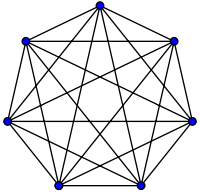# Complete graph

﻿
Complete graph
Complete graphK7, a complete graph with 7 vertices
Vertices n
Edges$\frac{n (n-1)}{2}$
Diameter 1
Girth 3 if n ≥ 3
Automorphisms n! (Sn)
Chromatic number n
Chromatic index n if n is odd
n − 1 if n is even
Spectral Gap n
Properties (n − 1)-regular
Symmetric graph
Vertex-transitive
Edge-transitive
Unit distance
Strongly regular
Integral
Notation Kn
v · mathematical field of graph theory, a complete graph is a simple undirected graph in which every pair of distinct vertices is connected by a unique edge.

## Properties

The complete graph on n vertices has n(n − 1)/2 edges (a triangular number), and is denoted by Kn (from the German komplett). It is a regular graph of degree n − 1. All complete graphs are their own maximal cliques. They are maximally connected as the only vertex cut which disconnects the graph is the complete set of vertices. The complement graph of a complete graph is an empty graph.

If the edges of a complete graph are each given an orientation, the resulting directed graph is called a tournament.

## Geometry and topology

A complete graph with n nodes represents the edges of an (n − 1)-simplex. Geometrically K3 forms the edge set of a triangle, K4 a tetrahedron, etc. The Császár polyhedron, a nonconvex polyhedron with the topology of a torus, has the complete graph K7 as its skeleton. Every neighborly polytope in four or more dimensions also has a complete skeleton.

K1 through K4 are all planar graphs. However, every planar drawing of a complete graph with five or more vertices must contain a crossing, and the nonplanar complete graph K5 plays a key role in the characterizations of planar graphs: by Kuratowski's theorem, a graph is planar if and only if it contains neither K5 nor the complete bipartite graph K3,3 as a subdivision, and by Wagner's theorem the same result holds for graph minors in place of subdivisions. As part of the Petersen family, K6 plays a similar role as one of the forbidden minors for linkless embedding.

## Examples

Complete graphs on n vertices, for n between 1 and 12, are shown below along with the numbers of edges: